# Pseudo-metric

(diff) ← Older revision | Latest revision (diff) | Newer revision → (diff)

on a setA non-negative real-valued functiondefined on the set of all pairs of elements of(that is, on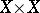) and subordinate to the following three restrictions, called the axioms for a pseudo-metric:

a) if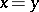, then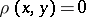;

b)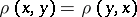;

c)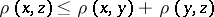, where,andare arbitrary elements of.

It is not required thatimplies. A topology onis determined as follows by a pseudo-metricon: A pointbelongs to the closure of a set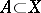if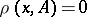, where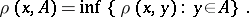This topology is completely regular but is not necessarily Hausdorff: singleton sets can be non-closed. Every completely-regular topology can be given by a collection of pseudo-metrics as the lattice union of the corresponding pseudo-metric topologies. Analogously, families of pseudo-metrics can be used in defining, describing and investigating uniform structures.

How to Cite This Entry:
Pseudo-metric. Encyclopedia of Mathematics. URL: http://encyclopediaofmath.org/index.php?title=Pseudo-metric&oldid=14367
This article was adapted from an original article by A.V. Arkhangel'skii (originator), which appeared in Encyclopedia of Mathematics - ISBN 1402006098. See original article